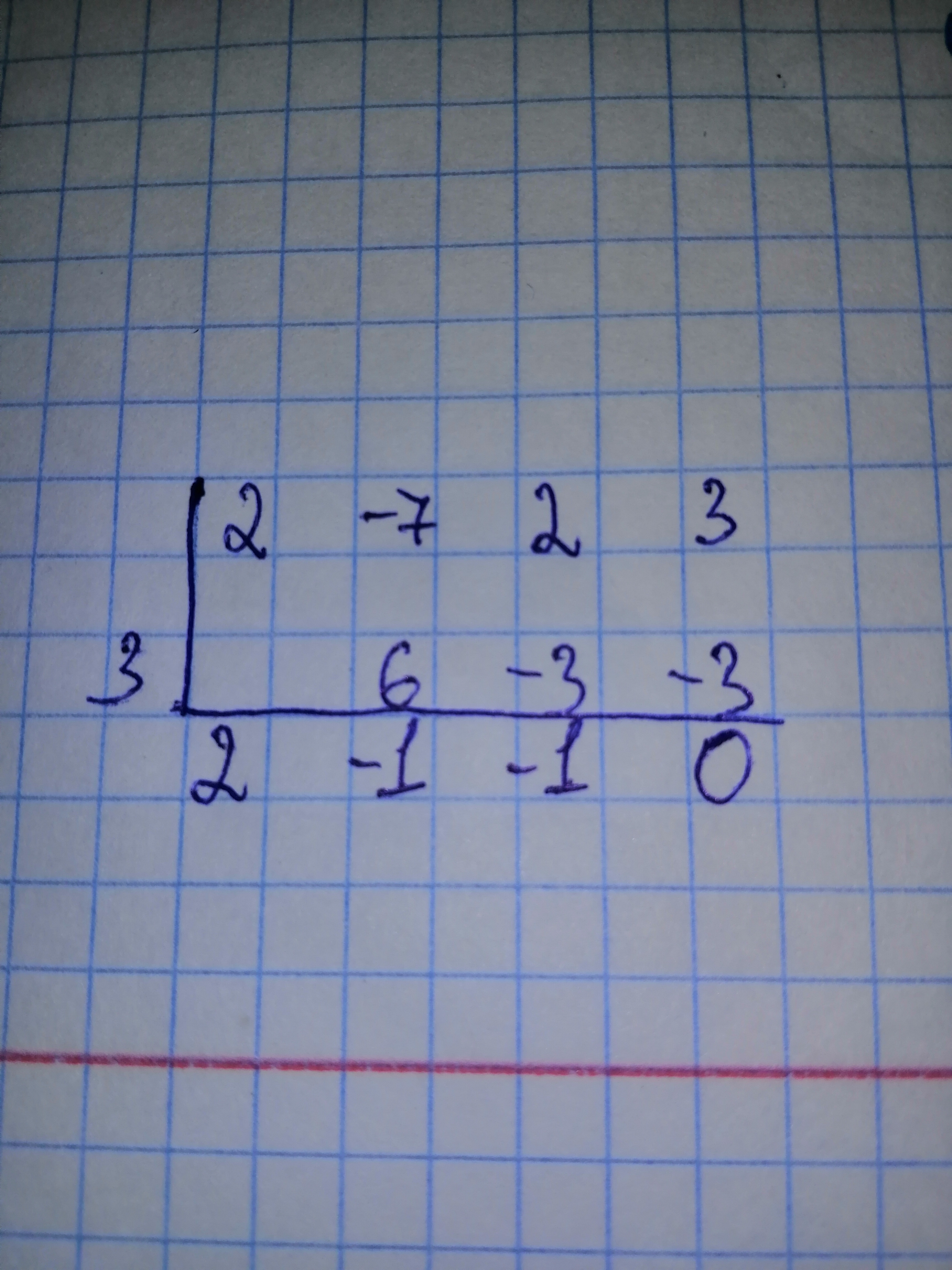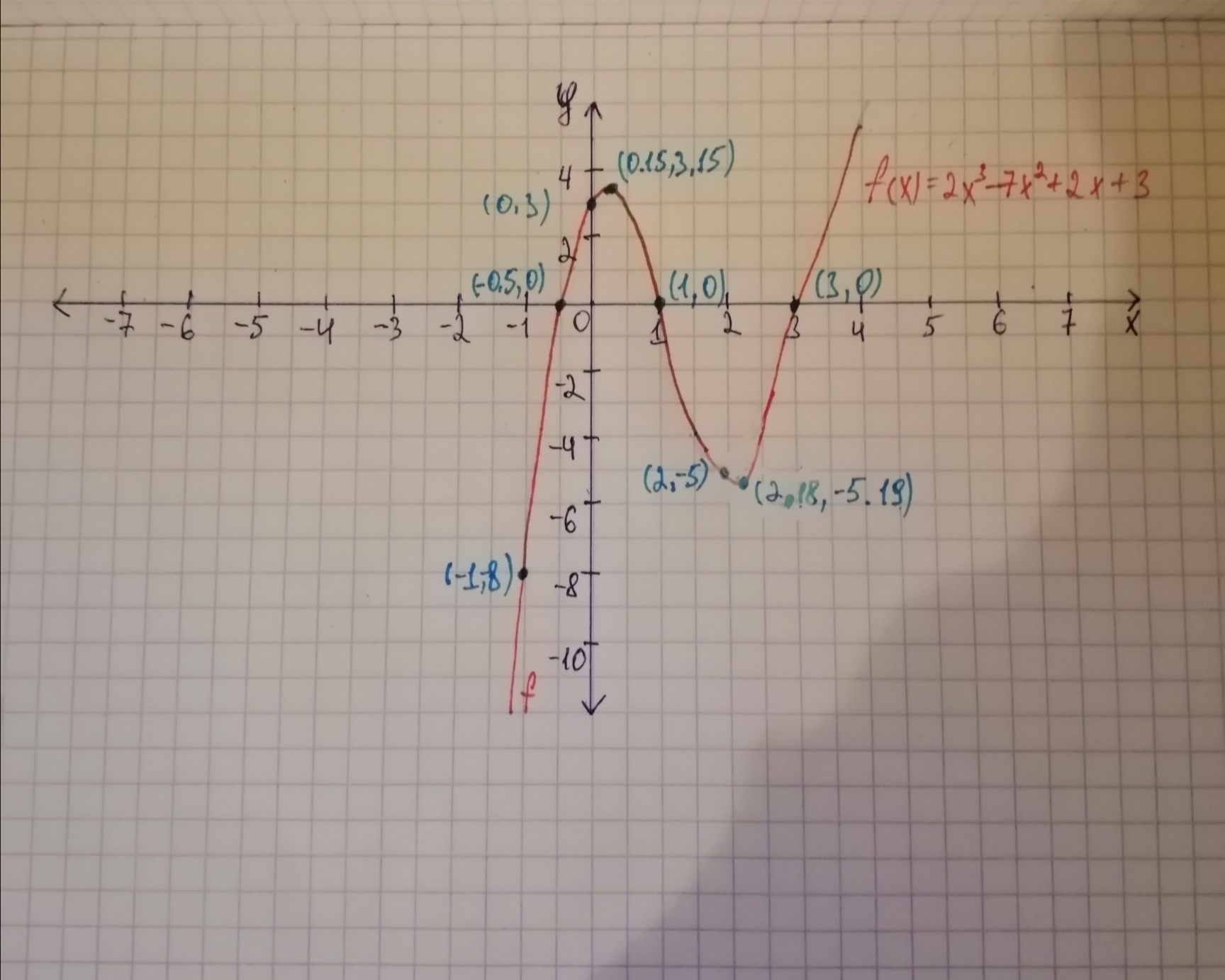# Graph each polynomial function.f(x)=2x^{3) - 7x^{2} + 2x + 3given that 3 is a zero.Tammy Todd 2021-02-05 Answered

Graph each polynomial function. given that 3 is a zero.

You can still ask an expert for help

• Questions are typically answered in as fast as 30 minutes

Solve your problem for the price of one coffee

• Math expert for every subject
• Pay only if we can solve itVelsenw

Step 1 To find the y-intercept evalute f(0)
Substitute 0 for x $=3$ Simplify So, the y-intercept is (0, 3)

Step 2 given that 3 is a zero Use synthetic divisionThe quotient is

So, are zeros of f(x) The graph of f(x) crosses the x-axis at Plot the points See the graph below.